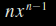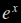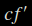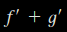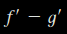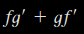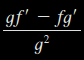# Stewart – Calculus – 3.2 – Differentiation Rules

THE PRODUCT RULE$\frac{d}{\mathit{dx}}[f(x)g(x)]=f(x)\frac{d}{\mathit{dx}}[g(x)]+g(x)\frac{d}{\mathit{dx}}[f(x)]$

(a) If$f(x)=xe^{x}$, find$f'(x)$.

(b) Find the nth derivative,$f^{(n)}(x)$.

Differentiate the function$f(t)=\sqrt{t}(a+\mathit{bt})$

If$f(x)=\sqrt{x} \hspace{1 mm} g(x)$, where$g(4)=2$ and$g'(4)=3$, find$f'(4)$.

THE QUOTIENT RULE$\frac{d}{\mathit{dx}}[\frac{f(x)}{g(x)}]=\frac{g(x)\frac{d}{\mathit{dx}}[f(x)]\text{--}f(x)\frac{d}{\mathit{dx}}[g(x)]}{[g(x)]^{2}}$

Let$y=\frac{x^{2}+x\text{--}2}{x^{3}+6}$

Find an equation of the tangent line to the curve$y=\frac{e^{x}}{(1+x^{2})}$ at the point$(1,\frac{1}{2}e)$.$\frac{d}{\mathit{dx}}(c)=?
$$\frac{d}{\mathit{dx}}(x^{n})=?
$$\frac{d}{\mathit{dx}}(e^{x})=?
$$(\mathit{cf})'=?
$$(f+g)'=?
$$(f-g)'=?
$$(\mathit{fg})'=?
$$(\frac{f}{g})'=?
$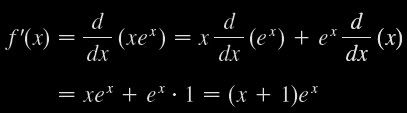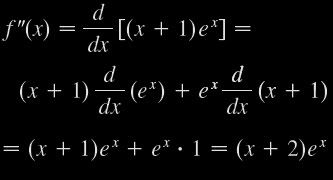SOLUTION 1
Using the Product Rule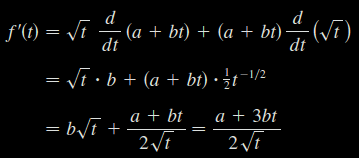SOLUTION 2
If we first use the laws of exponents to rewrite f(t), then we can proceed directly without using the Product Rule.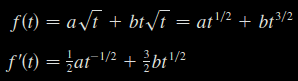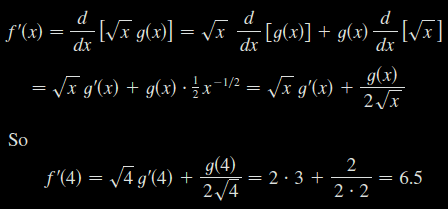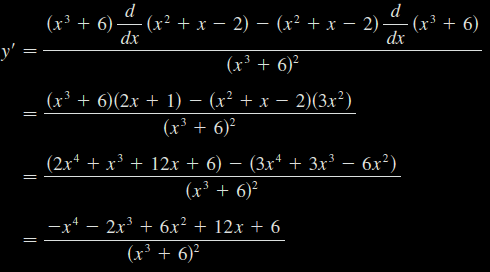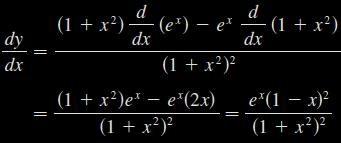0